# 基于停车信息的城市交通流量预测Urban Traffic Flow Prediction Based on Parking Information

• 全文下载: PDF(1994KB)    PP.397-404   DOI: 10.12677/OJTT.2018.76048
• 下载量: 260  浏览量: 745

In order to make effective use of parking information, this article uses sparking information ac-quisition system to obtain vehicle in and out data. On this basis, the “four-phase method” of traffic planning providing by TransCAD is applied to establish a macroscopic traffic planning model, and the dynamic traffic flow running state of the surrounding roads is predicted backwards. Finally, an urban traffic flow prediction method based on parking information is proposed, and it is proved that this method can effectively predict regional road network traffic flow.

1. 引言

2. 交通四阶段法

3. 基于停车信息的城市交通流量预测建模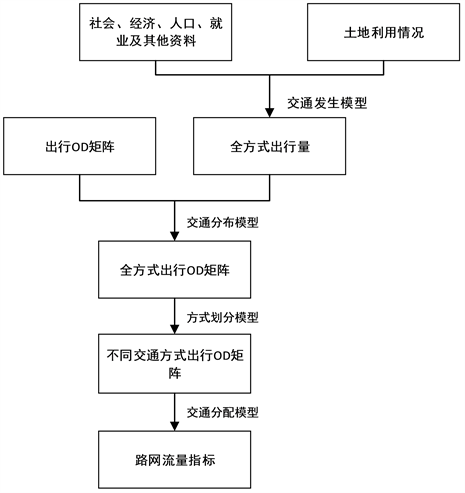Figure 1. Forecasting process

3.1. 出行分布

${q}_{ii}=K{O}_{i}^{\alpha }{D}_{i}^{\beta }{S}_{i}^{\gamma }$ (1)

$\alpha$$\beta$$\gamma$ 为待定系数

${q}_{ij}={A}_{i}{O}_{i}{B}_{j}{D}_{j}f\left({c}_{ij}\right)$ (2)

${A}_{i}=\frac{1}{\underset{j}{\sum }{B}_{j}{D}_{j}f\left({c}_{ij}\right)}$ (3)

${B}_{j}=\frac{1}{\underset{j}{\sum }{A}_{i}{O}_{i}f\left({c}_{ij}\right)}$ (4)

3.2. 交通分配

${p}_{ki}^{\omega }=\frac{{e}^{-{\theta }_{i}{c}_{k}^{\omega }}}{\underset{r\in {R}_{\omega }}{\sum }{e}^{-{\theta }_{i}{c}_{k}^{\omega }}},\forall k\in {R}_{\omega },\omega \in W,i\in I$ (5)

${q}_{\omega i}={D}_{\omega i}\left({C}_{\omega i}\right)\le {\stackrel{¯}{q}}_{\omega i},\forall \omega \in W,i\in I$ (6)

${C}_{\omega i}\left({c}_{\omega }\right)=E\left[\mathrm{min}\left(\underset{k\in {R}_{\omega }}{{C}_{ki}^{\omega }}\right)|{c}_{\omega }\right]=-\mathrm{ln}\underset{k\in {R}_{\omega }}{\sum }{e}^{-{\theta }_{i}{c}_{k}^{\omega }}/{\theta }_{i},\forall \omega \in W,i\in I$ (7)

${f}_{ki}^{\omega }={q}_{\omega i}{p}_{ki}^{\omega },\forall k\in {R}_{\omega },\omega \in W,i\in I$ (8)

4. 案例分析

4.1. 计算过程

1) 交通小区划分Table 1. Traffic cell data structure table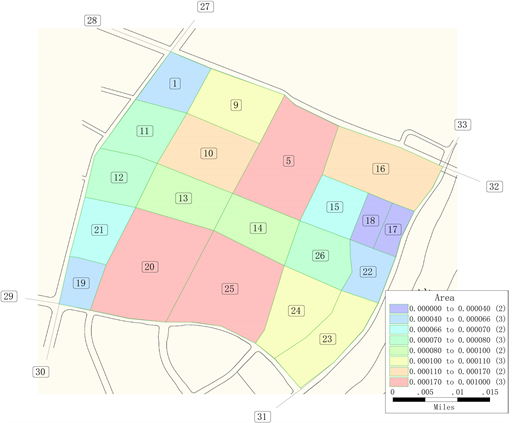Figure 2. Schematic diagram of traffic cell division

2) 建立路网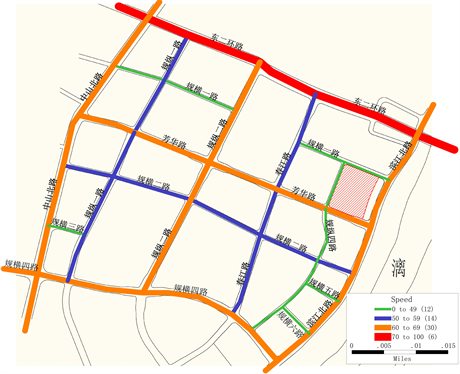Table 2. Road network data structure table

3) 交通分布Table 3. Study area travel OD matrix (1 - 10 cells)

4) 交通流分配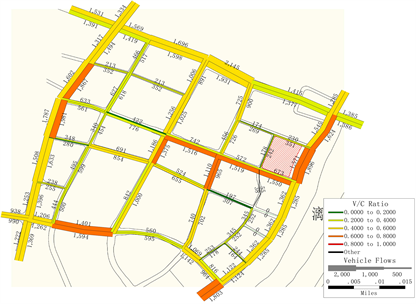Figure 4. Road section flow saturation map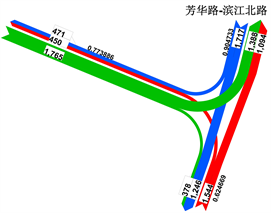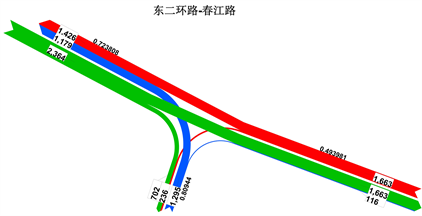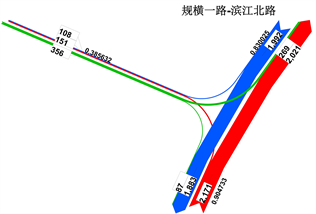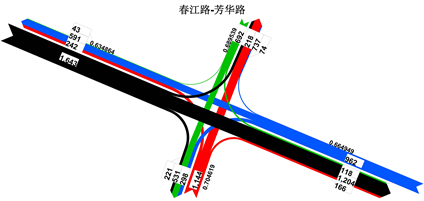Figure 5. Flow rate of intersections at part of the study area

4.2. 结果分析Table 4. Comparison of traffic volume prediction and distribution in some sections

5. 结语

  蒋潇, 王轶, 洪惠芬, 等. 住区困境: 人与车的挑战——城市住区停车问题的探究[J]. 中外建筑, 2011(12): 36-40.  关宏志, 王鑫, 王雪. 停车需求预测方法研究[J]. 北京工业大学学报, 2006, 32(7): 30-34.  郭学琴. 城市公共停车场规划研究[D]: [硕士学位论文]. 北京: 北京交通大学, 2007.  吴涛, 晏克非. 停车需求管理的机理研究[J]. 城市规划, 2002, 26(10): 85-88.  刘环宇. 基于TransCAD的轨道交通客流预测与分析[J]. 铁道建筑技术, 2014(s1): 278-279.  张磊, 刘小勇. 基于TransCAD进行交通需求预测的交通影响评价研究[J]. 交通运输研究, 2014(19): 44-48.